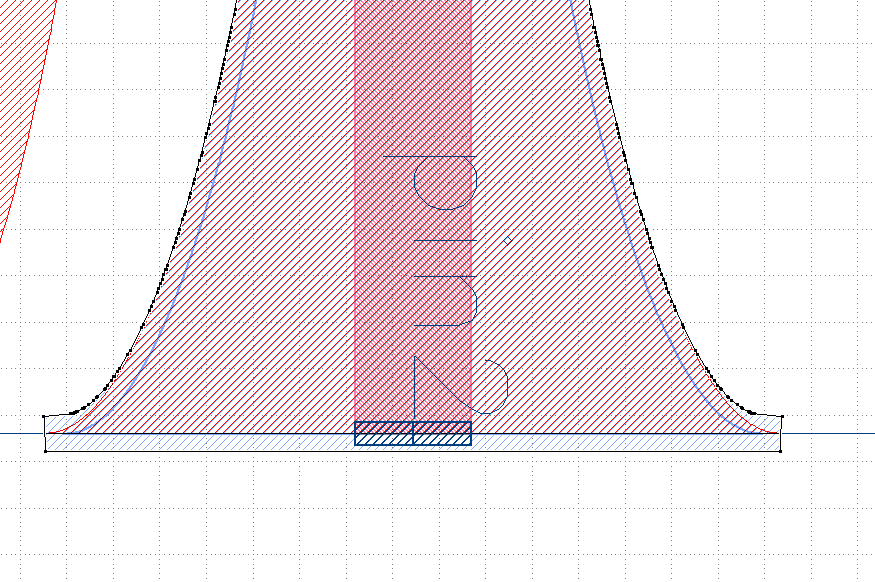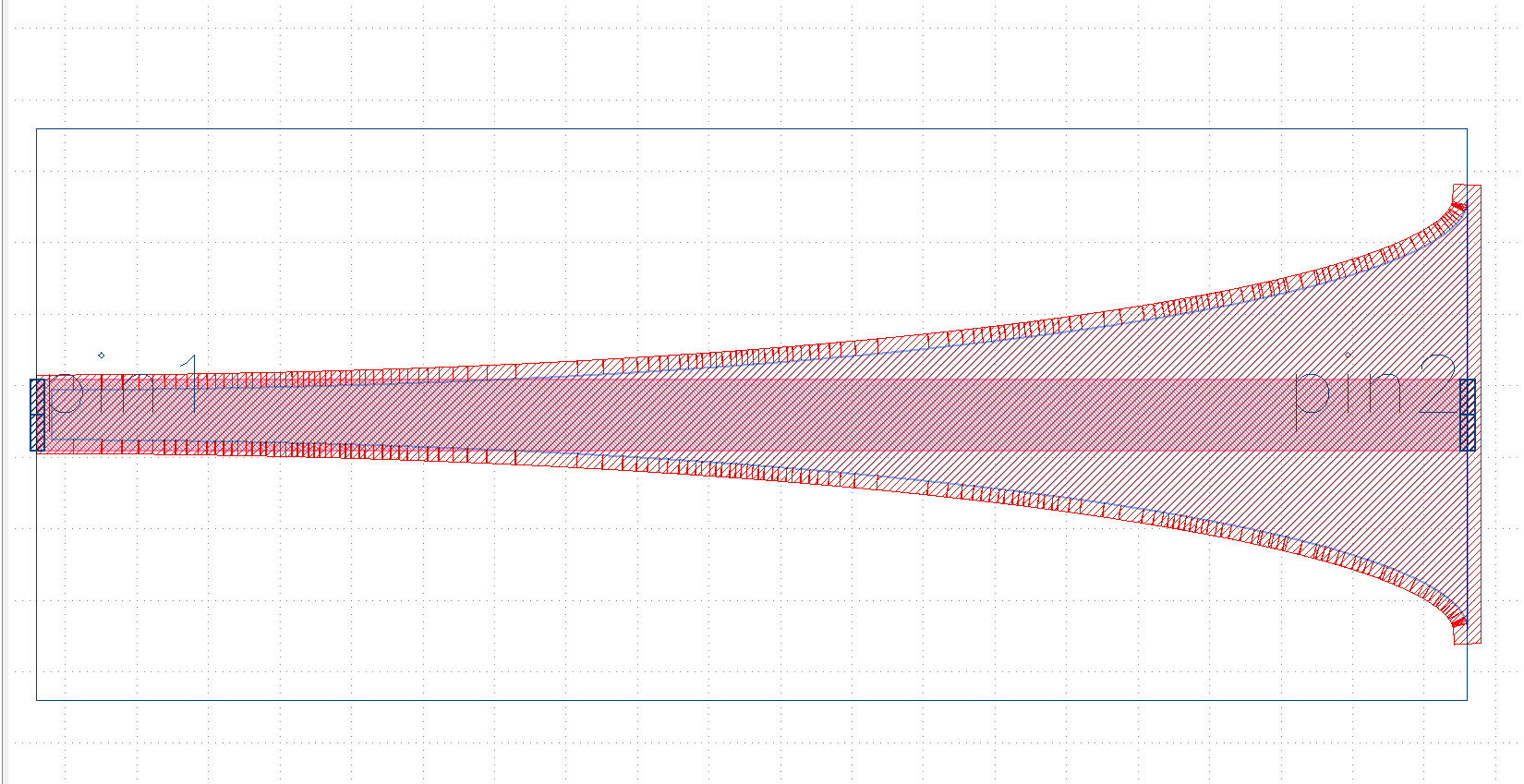How to size a polygon (make by points in array) by a fixed size?

edited June 2021

Hi,

I am trying to size a polygon, like what in "Edit->Selection->Size Shapes" for a polygon, but in Pcell? I have no idea about this.

The scalling function seems no function for this polygon,as it seems only work function for instantiate.The shape which need to size 0.1um is scripted by :

for i in range(len(x)):
pts.append(Point.from_dpoint(DPoint(x[i], y[i])))

# t = Trans(Trans.R0, x, 0)
# polygon = Polygon(pts,t)
polygon = Polygon(pts)
shapes(LayerSi901).insert(polygon)

Anyone who know how to size 0.1um for this polygon?

• You can cast the polygon into a Region using the constructor for Region which takes a polygon https://www.klayout.de/doc/code/class_Region.html

This would be something like sized_region = Region(polygon). You can then call the "size" function on the region sized_region.size(100). Note that you will need to scale the size to database units by dividing by your database unit. This can be obtained through the Layout.dbu parameter as detailed in the documentation: https://www.klayout.de/doc/code/class_Layout.html#method43

• Actually, I just noticed that the Polygon class has a "size" function, so it is as simple as

for i in range(len(x)):
pts.append(Point.from_dpoint(DPoint(x[i], y[i])))

# t = Trans(Trans.R0, x, 0)
# polygon = Polygon(pts,t)
polygon = Polygon(pts)
polygon.size(100)
shapes(LayerSi901).insert(polygon)

• edited June 2021

@Daaan Yes, polygon.size is there - but beware that it may generate strange "ears" and inner circuits. In order to remove them you'll need to merge the polygon which potentially generates multiple polygons or sometimes even eliminates all of them. So there is no single-polygon-to-single-polygon size function.

The Region class you mentioned is actually my preferred solution as it takes care of handling the size including the merge. It can handle a change in the number of polygons as it's actually a set of them.

Kind regards,

Matthias

• edited June 2021

Thank you for your answer. Both two methods can generate sized shape for a polygon.

As what Matthias said, the following way will apppear "ears", it acturally come up with some sharp points.

for i in range(len(x)):
pts.append(Point.from_dpoint(DPoint(x[i], y[i])))

# t = Trans(Trans.R0, x, 0)
# polygon = Polygon(pts,t)
polygon = Polygon(pts)
polygon.size(0.1/dbu)
shapes(LayerSi901).insert(polygon)The first method as what you suggest works well.

for i in range(len(x)):
pts.append(Point.from_dpoint(DPoint(x[i], y[i])))

sized_region = Region(polygon)
sized_region.size(0.1/dbu)
shapes(LayerSi901).insert(sized_region)

But I have another question, how to characterize a polygon when the 4 coordination of a polygon point are known, What is its grammar？

I tried those three method，they shows "No overload with matching arguments in Region.new in PCellDeclaration.produce\n .......".

polygon1 = [DPoint(length, -w4), DPoint(length, w4), DPoint(length + w4, w4), DPoint(length + w4, -w4)]
# polygon1 = [Point(length, -w4), Point(length, w4), Point(length + w4, w4), Point(length + w4, -w4)]
# polygon1 = [(length, -w4), (length, w4), (length + w4, w4), (length + w4, -w4)]
region2 = Region(polygon1)
shapes(LayerSi901).insert(polygon1)

If you know, could you tell me? It is difficult for me to get specific rules of writing polygon from this page.

Thank you very much.

• Your "polygon1" is not a Polygon type, it is an array of 'DPoints'. You must call the constructor for the Polygon, which takes an array of points. Something like the below (untested!) should work:

polygon1 = Polygon([DPoint(length, -w4), DPoint(length, w4), DPoint(length + w4, w4), DPoint(length + w4, -w4)])
region2 = Region(polygon1)
region2.size(0.1/dbu)
shapes(LayerSi901).insert(region2 )

I'm not sure if the DPoint array will work or if it must be strictly a Point array. In which case you may have to use something like:

polygon1 = Polygon([Point(length/dbu, -w4/dbu), Point(length/dbu, w4/dbu), Point(length/dbu + w4/dbu, w4/dbu), Point(length/dbu + w4/dbu, -w4/dbu)])

A "Region" is a collection of shapes and tends to be my preference when working with complex shapes. Regions can be combined (boolean), sized, smoothed, etc. Note that the Region can take a Polygon as a parameter for creation. You may then resize the Region, and pass that to the insert() function of your cell. I hope that is reasonably clear?

Both methods (DPoint,Point) constructing polygon works well.

# polygon1 = Polygon([DPoint(length, -w4), DPoint(length, w4), DPoint(length + w4, w4), DPoint(length + w4, -w4)])
polygon1 = Polygon([Point(length, -w4), Point(length, w4), Point(length + w4, w4), Point(length + w4, -w4)])
region2 = Region(polygon1)
# region2.size(0.1 / dbu)
shapes(LayerSi).insert(region2)

Beside, I find that both "polygon" and "region" can all be insert in shape.
Thank you again for your respond.

• @Daaan Yes, thanks for mentioning this. Region only works with integer-type objects. So that's Point, Polygon, Vector, Box, ... everything is in multiples of DBU.

Matthias